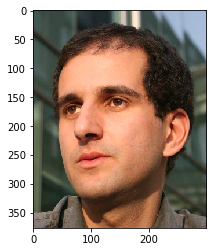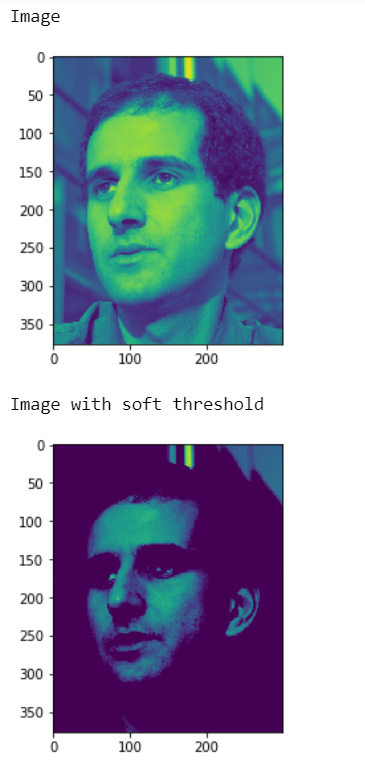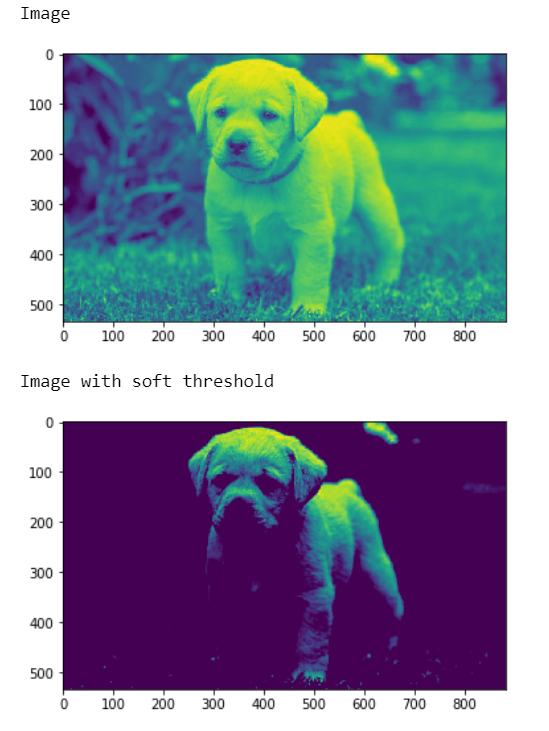Open in App
Not now

# Mahotas – Soft Threshold

• Last Updated : 01 Dec, 2021

In this article we will see how we can implement soft threshold in mahotas. The soft thresholding is also called wavelet shrinkage, as values for both positive and negative coefficients are being “shrinked” towards zero, in contrary to hard thresholding which either keeps or removes values of coefficients.

In this tutorial we will use “luispedro” image, below is the command to load it.

`mahotas.demos.load('luispedro')`

Below is the luispedro imageIn order to do this we will use mahotas.rc method

Syntax : mahotas.thresholding.soft_threshold(image, t_value)
Argument : It takes image object and unit8 value as argument
Return : It returns image object

Note : Input image should be filtered or should be loaded as grey

In order to filter the image we will take the image object which is numpy.ndarray and filter it with the help of indexing, below is the command to do this

`image = image[:, :, 0]`

Example 1:

## Python3

 `# importing required libraries``import` `mahotas``import` `mahotas.demos``import` `numpy as np``from` `pylab ``import` `imshow, gray, show``from` `os ``import` `path` `# loading the image``photo ``=` `mahotas.demos.load(``'luispedro'``)`  `# loading image as grey``photo ``=` `mahotas.demos.load(``'luispedro'``, as_grey ``=` `True``)` `# converting image type to unit8``# because as_grey returns floating values``photo ``=` `photo.astype(np.uint8)` `# showing original image``print``(``"Image"``)``imshow(photo)``show()` `# unit 8 value``t ``=` `np.uint8(``150``)` `# soft threshold``photo ``=` `mahotas.thresholding.soft_threshold(photo, t)`  `print``(``"Image with soft threshold"``)` `# showing image``imshow(photo)``show()`

Output :Example 2:

## Python3

 `# importing required libraries``import` `mahotas``import` `numpy as np``from` `pylab ``import` `imshow, show``import` `os`  `# loading image``img ``=` `mahotas.imread(``'dog_image.png'``)``    `  `# setting filter to the image``img ``=` `img[:, :, ``0``]` `print``(``"Image"``)` `# showing the image``imshow(img)``show()` `# unit 8 value``t ``=` `np.uint8(``180``)` `# soft threshold``img ``=` `mahotas.thresholding.soft_threshold(img, t)`  `print``(``"Image with soft threshold"``)` `# showing image``imshow(img)``show()`

Output :My Personal Notes arrow_drop_up